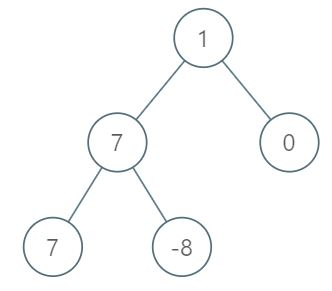# LeetCode解题分享：1161. Maximum Level Sum of a Binary Tree

##### Problem

Given the root of a binary tree, the level of its root is 1, the level of its children is 2, and so on.

Return the smallest level X such that the sum of all the values of nodes at level X is maximal.

Example 1:Input: [1,7,0,7,-8,null,null]
Output: 2
Explanation:
Level 1 sum = 1.
Level 2 sum = 7 + 0 = 7.
Level 3 sum = 7 + -8 = -1.
So we return the level with the maximum sum which is level 2.

Note:

The number of nodes in the given tree is between 1 and 10^4.
-10^5 <= node.val <= 10^5

##### 解题思路

标准的层次遍历二叉树。

代码如下：

# Definition for a binary tree node.
# class TreeNode:
#    def __init__(self, x):
#        self.val = x
#        self.left = None
#        self.right = None

class Solution:
def maxLevelSum(self, root: TreeNode) -> int:
q = collections.deque()

q.append(root)

max_level = None
max_sum = - float("inf")

level = 1

while q:
length = len(q)
sum = 0
for i in range(length):
node = q.popleft()
sum += node.val

if node.left:
q.append(node.left)

if node.right:
q.append(node.right)

if sum > max_sum:
max_sum = sum
max_level = level

level += 1

return max_level